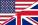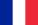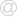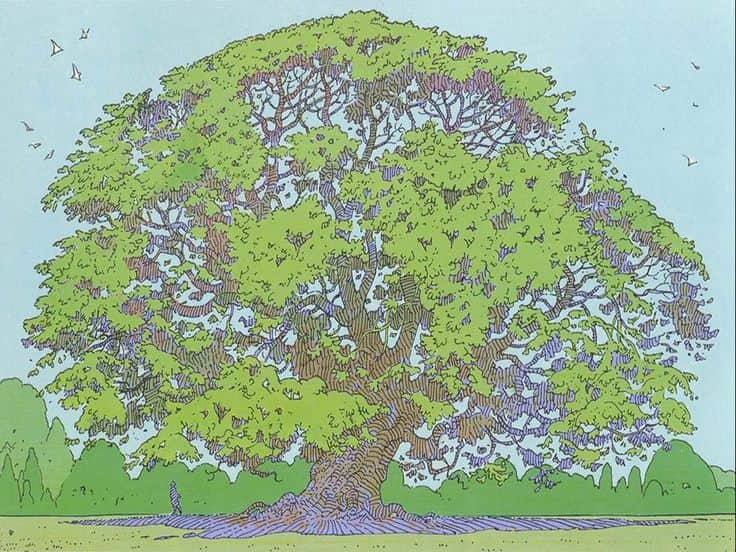# Lorenzo Fantini

### École PolytechniqueI am a Monge Professor at the Centre de Mathématiques Laurent Schwartz of the École Polytechnique.

My pronouns are he/him/his.

Centre de Mathématiques Laurent Schwartz
École Polytechnique
91128 Palaiseau Cedex - France

Office: 06.1011

Email: lorenzo.fantinipolytechnique.edu

## ResearchA Berkovich curve drawn by Mœbius.
I'm interested in algebraic geometry.
More specifically, I like to apply non-archimedean analytic geometry, especially from the point of view of Berkovich, to problems in birational geometry (singularity theory, Lipschitz geometry, motivic integration), arithmetic geometry (models of curves and ramification), and combinatorics (tropical geometry).A Berkovich curve drawn by Mœbius.

### Preprints

1. "On Lipschitz normally embedded singularities" (with A. Pichon)
– submitted, 18 pages, 2022 (arXiv)
Any subanalytic germ (X,0) in (ℝn,0) is equipped with two natural metrics: its outer metric, induced by the standard Euclidean metric of the ambient space, and its inner metric, which is defined by measuring the shortest length of paths on the germ (X,0). The germs for which these two metrics are equivalent up to a bilipschitz homeomorphism, which are called Lipschitz Normally Embedded, have attracted a lot of interest in the last decade. In this survey we discuss many general facts about Lipschitz Normally Embedded singularities, before moving our focus to some recent developments on criteria, examples, and properties of Lipschitz Normally Embedded complex surfaces. We conclude the manuscript with a list of open questions which we believe to be worth of interest.

### Publications

1. "Triangulations of non-archimedean curves, semi-stable reduction, and ramification" (with D. Turchetti)
– Annales de l'Institut Fourier, 73(2), 695–746, 2023 (arXiv, DOI)
Let K be a complete discretely valued field with algebraically closed residue field and let C be a smooth projective and geometrically connected algebraic K-curve of genus g. Assume that g≥2, so that there exists a minimal finite Galois extension L of K such that CL admits a semi-stable model. In this paper, we study the extension L|K in terms of the minimal triangulation of Can, a distinguished finite subset of the Berkovich analytification of C. We prove that the least common multiple d of the multiplicities of the points of the minimal triangulation always divides the degree [L:K]. Moreover, if d is prime to the residue characteristic of K, then we show that d=[L:K], obtaining a new proof of a classical theorem of T. Saito on tame ramification. We then discuss curves with marked points, which allows us to prove analogous results in the case of elliptic curves, whose minimal triangulations we describe in full in the tame case. In the last section, we illustrate through several examples how our results explain the failure of the most natural extensions of Saito's theorem to the wildly ramified case.
2. "Polar exploration of complex surface germs" (with A. Belotto da Silva, A. Némethi, and A. Pichon)
– Transactions of the American Mathematical Society, 375(9), 6747–6767, 2022 (arXiv, DOI)
We prove that the topological type of a normal surface singularity (X,0) provides finite bounds for the multiplicity and polar multiplicity of (X,0), as well as for the combinatorics of the families of generic hyperplane sections and of polar curves of the generic plane projections of (X,0). A key ingredient in our proof is a topological bound of the growth of the Mather discrepancies of (X,0), which allows us to bound the number of point blowups necessary to achieve factorization of any resolution of (X,0) through its Nash transform. This fits in the program of polar explorations, the quest to determine the generic polar variety of a singular surface germ, to which the final part of the paper is devoted.
3. "On Lipschitz normally embedded complex surface germs" (with A. Belotto da Silva and A. Pichon)
– Compositio Mathematica, 158(3), 623–653, 2022 (arXiv, DOI)
We undertake a systematic study of Lipschitz Normally Embedded normal complex surface germs. We prove in particular that the topological type of such a germ determines the combinatorics of its minimal resolution which factors through the blowup of its maximal ideal and through its Nash transform, as well as the polar curve and the discriminant curve of a generic plane projection, thus generalizing results of Spivakovsky and Bondil that were known for minimal surface singularities. In an appendix, we give a new example of a Lipschitz Normally Embedded surface singularity.
4. "Inner geometry of complex surfaces: a valuative approach" (with A. Belotto da Silva and A. Pichon)
– Geometry & Topology, 26(1), 163–219, 2022 (arXiv, DOI)
Bonus : here are the slides for a 15 minutes long talk I gave about the main result of this paper.
Given a complex analytic germ (X,0) in (ℂn,0), the standard Hermitian metric of n induces a natural arc-length metric on (X,0), called the inner metric. We study the inner metric structure of the germ of an isolated complex surface singularity (X,0) by means of a family of natural numerical invariants, called inner rates. Our main result is a formula for the Laplacian of the inner rate function on a space of valuations, the non-archimedean link of (X,0). We deduce in particular that the global data consisting of the topology of (X,0), together with the configuration of a generic hyperplane section and of the polar curve of a generic plane projection of (X,0), completely determine all the inner rates on (X,0), and hence the local metric structure of the germ. Several other applications of our formula are discussed in the paper.
5. "Motivic and analytic nearby fibers at infinity and bifurcation sets" (with M. Raibaut)
– Arc Schemes and Singularities, World Scientific Publishing, 197–220, 2020. (arXiv, DOI)
In this paper we use motivic integration and non-archimedean analytic geometry to study the singularities at infinity of the fibers of a polynomial map f: 𝔸d → 𝔸1. We show that the motivic nearby cycles at infinity Sf,a of f for a value a is a motivic generalization of the classical invariant λf(a), an integer that measures a lack of equisingularity at infinity in the fiber f-1(a). We then introduce a non-archimedean analytic nearby fiber at infinity Ff,a whose motivic volume recovers the motive Sf,a. With Sf,a and Ff,a can be naturally associated a motivic and an analytic bifurcation sets respectively; we show that the first one always contains the second, and that both contain the classical topological bifurcation set of f if f has isolated singularities at infinity.
6. "Links of sandwiched surface singularities and self-similarity" (with C. Favre and M. Ruggiero)
– Manuscripta Mathematica, 162(1-2), 23–65, 2020 (arXiv, DOI)
We characterize sandwiched singularities in terms of their link in two different settings. We first prove that such singularities are precisely the normal surface singularities having self-similar non-archimedean links. We describe this self-similarity both in terms of Berkovich analytic geometry and of the combinatorics of weighted dual graphs. We then show that a complex surface singularity is sandwiched if and only if its complex link can be embedded in a Kato surface in such a way that its complement remains connected.
7. "Galois descent of semi-affinoid spaces" (with D. Turchetti)
– Mathematische Zeitschrift, 290(3-4), 1085–1114, 2018 (arXiv, DOI)
We study the Galois descent of semi-affinoid non-archimedean analytic spaces. These are the non-archimedean analytic spaces which admit an affine special formal scheme as model over a complete discrete valuation ring, such as for example open or closed polydiscs or polyannuli. Using Weil restrictions and Galois fixed loci for semi-affinoid spaces and their formal models, we describe a formal model of a K-analytic space X, provided that XKL is semi-affinoid for some finite tamely ramified extension L of K. As an application, we study the forms of analytic annuli that are trivialized by a wide class of Galois extensions that includes totally tamely ramified extensions. In order to do so, we first establish a Weierstrass preparation result for analytic functions on annuli, and use it to linearize finite order automorphisms of annuli. Finally, we explain how from these results one can deduce a non-archimedean analytic proof of the existence of resolutions of singularities of surfaces in characteristic zero.
8. "Normalized Berkovich spaces and surface singularities"
– Transactions of the American Mathematical Society, 370(11), 7815–7859, 2018 (arXiv, DOI)
We define normalized versions of Berkovich spaces over a trivially valued field k, obtained as quotients by the action of ℝ>0 defined by rescaling semivaluations. We associate such a normalized space to any special formal k-scheme and prove an analogue of Raynaud's theorem, characterizing categorically the spaces obtained in this way. This construction yields a locally ringed G-topological space, which we prove to be G-locally isomorphic to a Berkovich space over the field k((t)) with a t-adic valuation. These spaces can be interpreted as non-archimedean models for the links of the singularities of k-varieties, and allow to study the birational geometry of k-varieties using techniques of non-archimedean geometry available only when working over a field with non-trivial valuation. In particular, we prove that the structure of the normalized non-archimedean links of surface singularities over an algebraically closed field k is analogous to the structure of non-archimedean analytic curves over k((t)), and deduce characterizations of the essential and of the log essential valuations, i.e. those valuations whose center on every resolution (respectively log resolution) of the given surface is a divisor.
9. "Faithful realizability of tropical curves" (with M. Cheung, J. Park, and M. Ulirsch)
– International Mathematics Research Notices, 2016(15), 4706–4727, 2016 (arXiv, DOI)
We study whether a given tropical curve Γ in ℝn can be realized as the tropicalization of an algebraic curve whose non-archimedean skeleton is faithfully represented by Γ. We give an affirmative answer to this question for a large class of tropical curves that includes all trivalent tropical curves, but also many tropical curves of higher valence. We then deduce that for every metric graph G with rational edge lengths there exists a smooth algebraic curve in a toric variety whose analytification has skeleton G, and the corresponding tropicalization is faithful. Our approach is based on a combination of the theory of toric schemes over discrete valuation rings and logarithmically smooth deformation theory, expanding on a framework introduced by Nishinou and Siebert.
10. "Normalized non-archimedean links and surface singularities"
– Comptes Rendus Mathématique, 352(9), 719–723, 2014 (Pdf, DOI)
This note announced some of the results of the paper "Normalized Berkovich spaces and surface singularities".

## Teaching

MAA306 - Differential Geometry (2023-2024) on Moodle.

MAT451 - Algèbre et Théorie de Galois (2023-2024) on Moodle.

I am the Academic Advisor in charge of the first year Math curriculum of the Bachelor of Science of École Polytechnique. Students looking for information should find it on Moodle, SynapseS, or get in touch with me by email.

## Curriculum Vitae

#### 2021-today: Professeur Monge

École Polytechnique (France).

#### 2019-2021: Research Fellow

Goethe Universität Frankfurt (Germany), in the group of Annette Werner. Supported by the Alexander von Humboldt Foundation.

#### 2018-2019: Postdoctoral fellow

Aix-Marseille Université (France), ANR LISA.

#### 2016-2018: Postdoctoral fellow

Sorbonne Université (France), ANR DEFIGEO.

#### 2014-2016: Postdoctoral fellow

École Polytechnique (France), in the group of Charles Favre.

#### 2010-2014: Doctoral fellow

University of Leuven (Belgium).

My PhD advisor was Johannes Nicaise.

#### 2008-2010: Erasmus Mundus Master ALGANT.

I spent the first year of my Master degree at the University of Padova (Italy) and the second one at the University of Paris-Sud, Orsay (France).

If you want to find out more about me, download a complete CV (also available in French).

I'm collecting here some links, mainly for my convenience. I share them in case they might be useful to someone else.

ANR SINTROP

GDR Singularités et Applications

### Seminars

Tropical Geometry in Frankfurt (TGiF), a seminar series on tropical geometry which I used to organize together with Martin Ulirsch, is still taking place online. Information about the upcoming sessions can be found here.

Here is a (rather old) list of some interesting seminars taking place in or around Paris:

### Upcoming events

And here are some upcoming events that caught my eye. I will most likely attend only a few of them:

Calendar of events at CIRM, Luminy: 2023, 2024.

Lists of conferences:

Some past events I attended are archived here.

### Videos

There has never been a better time to watch some recorded math talks. Lots of videos of seminars can be found on the following pages. These should keep us busy for a while, but if you now of any other good sources please let me know and I'll add them to the list.
And one site to rule them all:

Here I uploaded a couple old papers that I had some trouble finding or I had to scan myself. Hopefully search engines will index them and this will save other people some time.

I strongly believe in Federico Ardila's axioms:

1. Mathematical potential is distributed equally among different groups, irrespective of geographic, demographic, and economic boundaries.
2. Everyone can have joyful, meaningful, and empowering mathematical experiences.
3. Mathematics is a powerful, malleable tool that can be shaped and used differently by various communities to serve their needs.
4. Every student deserves to be treated with dignity and respect.